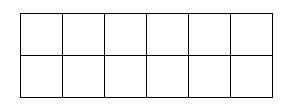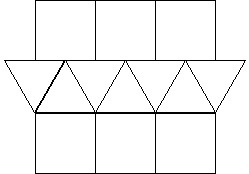## Shapes, Symmetry & Tessellation

Symmetry

If a shape has a line of symmetry, the line of symmetry will divide the shape into two equal parts, one half of which can be folded along the line of symmetry to fit exactly onto the other. Note, a rectangle has two (not four) lines of symmetry and a circle has an infinite number.

If rotating a shape through a certain angle produces an identical shape, it has rotational symmetry. If the shape can be rotated 4 times before returning to its original shape (e.g. a square), it has "rotational symmetry of order 4". An equilateral triangle has rotational symmetry of order 3 and a rectangle has rotational symmetry of order 2.

Triangles

Isosceles triangles have two equal angles. The sides of the triangle opposite the equal angles are equal in length to one another.
Equilateral triangles have all of their sides and angles equal. Since there are 180 degrees in a triangle and all the angles are equal, each angle is 60 degrees.

Other Shapes

A polygon is any shape with straight sides. A regular polygon is where all of the interior angles are the same.

• Parallelogram: opposite sides are parallel, opposite angles are equal, the diagonals bisect one another (this means the diagonals cross at their midpoints).
• Rhombus: (a parallelogram with all four sides of equal length), diagonals bisect one another at right angles.
• Trapezium: One pair of opposite sides are parallel.
• Square: All sides are equal, all angles are 90 degrees, diagonals bisect one another at 90 degrees.
• Rectangle: All angles are 90 degrees, diagonals bisect one another.

Tessellation

A shape is said to tessellate if an infinite number of that shape can be put together, leaving no gaps. For example, a square tessellates:Other shapes which tessellate include equilateral triangles and hexagons. Shapes can also tessellate with one another, for example equilateral triangles and squares tessellate with one another: